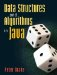# A.5. Branching

We often want our programs to make decisions based on the value of some variable. Java has two statements for this: if and switch.

The if statement allows us to execute a body only if some test evaluates to true. In Figure A-6, we wrote a do loop which was not exactly equivalent to the while and for loop examples from the same section. The difference is that, if args.length happens to be exactly zero (that is, no command-line arguments are provided), the do loop executes its body once anyway. This causes the program to crash, because line 5 tries to refer to args, and there is no such element. We can fix this with anif statement (Figure A-10).

Figure A-10. With an added if, the do loop becomes exactly equivalent to the while and for loops.

 ``` 1 /** Print a friendly greeting. */ 2 public static void main(String[] args) { 3 if (args.length > 0) { 4 int index = 0; 5 do { 6 System.out.println("Hello, " + args[index] + "!"); 7 index = index + 1; 8 } while (index < args.length); 9 } 10 } ```

Optionally, an if statement can end with the keyword else followed by another body. This body is executed if the test evaluates to false. We can deal with several different possibilities with a statement of this form:

```if (...) {
...
} else if (...) {
...
} else if (...) {
...
} else {
...
}
```

This is illustrated in the Guess program (Figure A-11). The if statement beginning on line 15 first tests whether the variable comparison is the char '<'. If so, the program adjusts the range of numbers it will consider for its next guess.

Figure A-11. A first draft of the Guess program. A do loop is appropriate here, because the program always has to make at least one guess. The statement on line 14 reads the next line from the user, extracts the first character, and stores it in the variable comparison.

 ``` 1 /** Game where the computer guesses a number. */ 2 public class Guess { 3 4 /** Play the game. */ 5 public static void main(String[] args) { 6 java.util.Scanner input = new java.util.Scanner(System.in); 7 System.out.println("Think of an integer between 1 and 100."); 8 int min = 1; 9 int max = 100; 10 char comparison; 11 do { 12 int guess = min; 13 System.out.print("Is it <, =, or > than " + guess + "? "); 14 comparison = input.nextLine().charAt(0); 15 if (comparison == '<') { 16 max = guess - 1; 17 } else if (comparison == '=') { 18 System.out.println("I win!"); 19 } else { 20 min = guess + 1; 21 } 22 } while (comparison != '='); 23 } 24 25 } 26 ```

Otherwise, the program tests whether comparison is '='. If so, the program declares victory. Otherwise, it assumes that comparision must be '>'.

An equivalent way to write this program is using a switch statement (Figure A-12). This checks for various possible values of one variable. The code from the first matching case up to the next break statement (or the end of the switch statement) is executed.

Figure A-12. The same loop using a switch statement.

 ``` 1 do { 2 int guess = min; 3 System.out.print("Is it <, =, or > than " + guess + "? "); 4 comparison = input.nextLine().charAt(0); ``` 5 switch (comparison) { 6 case '<': 7 max = guess - 1; 8 break; 9 case '=': 10 System.out.println("I win!"); 11 break; 12 default: 13 min = guess + 1; 14 } 15 } while (comparison != '=');

A handy feature of the switch statement is that, if we don't put a break statement at the end of a case, Java will keep right on going to the next one. For example, suppose that while running the program we keep accidentally typing a comma when we mean to type a less-than sign. We can add an extra case for this which prints a message and then treats the comparison as '<' (Figure A-13).

Figure A-13. After executing its own code, the top case falls through to the following one.

 ``` 1 switch (comparison) { 2 case ',': 3 System.out.println("I assume you meant '<'."); 4 case '<': 5 max = guess - 1; 6 break; 7 case '=': 8 System.out.println("I win!"); 9 break; 10 default: 11 min = guess + 1; 12 } ```

Exercises

 A.12 Modify the program in Figure A-11 so that it behaves the same way, but does not contain the keyword else. (Hint: The first occurrence of else, on line 17, can simply be replaced with a newline. Why doesn't this work for the one on line 19?)Data Structures and Algorithms in Java
ISBN: 0131469142
EAN: 2147483647
Year: 2004
Pages: 216
Authors: Peter Drake